omG8HI9LSKFW6lhCj8prTs0Z6lUhdOT9Jhi1Sf4m

# Maximum Power Transfer Theorem

In many practical situations, a circuit is designed to provide power to a load. There are applications in areas such as communications where it is desirable to maximize the power delivered to a load. We now address the problem of delivering the maximum power to a load when given a system with known internal losses. It should be noted that this will result in significant internal losses greater than or equal to the power delivered to the load.

This circuit analysis theorems are classified as:
1. Superposition theorem
2. Source transformation
3. Thevenin theorem
4. Norton theorem
5. Maximum power transfer

## Maximum Power Transfer Theory

The Thevenin equivalent is useful in finding the maximum power a linear circuit can deliver to a load. We assume that we can adjust the load resistance RL.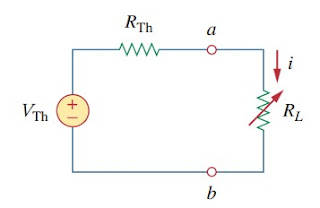Figure 1
If the entire circuit is replaced by its Thevenin equivalent except for the load, as shown in Figure.(1), the power delivered to the load is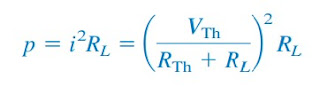(1)
For a given circuit, VTh and RTh are fixed. By varying the load resistance RL, the power delivered to the load varies as sketched in Figure.(2). We notice from Figure.(2) that the power is small for small or large values of RL but maximum for some value of RL between 0 and ∞.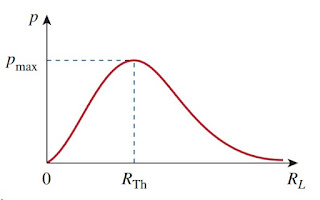Figure 2
Let me show that this maximum power occurs when RL is equal to RTh. This is known as the maximum power theorem.
Maximum power is transferred to the load when the load resistance equals the Thevenin resistance as seen from the load (RL = RTh)
To prove the maximum power transfer theorem, we differentiate p in Equation.(1) with the respect to RL and set the result equal to zero. We obtain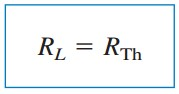(3)
showing that the maximum power transfer takes place when the load resistance RL equals the Thevenin resistance RTh. We can readily confirm that Equation.(3) gives the maximum power by showing that d2p/dRL2<0.

The maximum power transferred is obtained by susbtituting Equations.(3) to (1), for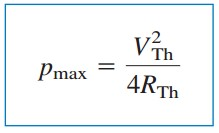(4)
Equation.(4) applies only when R= RTh. When R≠ RTh, we compute the power delivered to the load using Equation.(1).

## Maximum Power Transfer Example

For better understanding, let us review the example below :
1.Find the value of RL for maximum power transfer in the circuit of Figure.(3)
We need to find the Thevenin resistance RTh and the Thevenin voltage VTh across the terminals a-b. To get RTh, we use the circuit in Figure.(4a) and obtain
Solving for i1, we get i1 = -2/3. Applying KVL around the outer loop to get VTh across terminals a-b, we obtain
Related Posts
SHARE